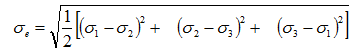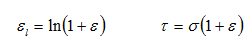# Plasticity Simulation Tips & TricksAugust 14, 2015 By: Peter Barrett

Linear elastic material modeling is an excellent assumption for structures that undergo small deformations and return to their original shape upon removal of load.  Using an elastic modulus and Poisson's ratio for the initial simulation is a good practice for all analyses, since it solves in a single iteration and provides benchmark stress results.  However, many materials undergo permanent deformation, even under design load conditions, which make the linear assumption invalid. With metallic structures, permanent deformations will occur when the Von Mises yield stress is exceeded, and the resulting plastic strains can often become significant.

The permanent displacements are a result of  the dissipation of energy and are irreversible, which makes the event path dependent.  Thus, analytical simulations require the load stepping to follow the real loading sequence. Although the theory of plasticity grew out of the study of metals, one can often apply bilinear or multi-linear isotropic hardening laws to many other materials, as long as the underlying theory and analysis is applicable.

Plasticity modeling can be subdivided into:

• Loadings that induce only small plastic strains, which are roughly the same order as the elastic strains, where cyclic loading and a kinematic hardening law should be utilized.
• Very large strains and deformations, so large that the elastic strains can be disregarded and ultimate capacity is typically the design concern, where an isotropic hardening law is needed.

Plastic deformations can also be either rate independent or rate dependent, but this blog is limited to rate independent discussions.  A typical plasticity material response of stress vs. strain (equal to force vs. displacement for a tensile test) is illustrated in Figure 1.

Figure 1 Uniaxial Stress (Force) vs. Strain (Displacement) Response

## plasticity1.pngIn nearly all FEA software, the three-dimensional stress state is converted for purposes of material yield evaluation to an equivalent uniaxial stress state using the Von Mises Yield Criterion.When large displacements are active, the finite element analysis accounts for the reduction in cross-sectional area under increased load. Thus the material model must be consistent with this change in geometry. This is achieved by converting nominal stress and engineering strain data typically output from a tensile test into true stress and log strain values utilizing the following formulas.Often, the input needed for a multilinear stress-strain law requires the true stress vs. plastic strain instead of the total strain described above. In this case, the first data point should be zero strain and the yield stress.  A common mistake made in entering the remaining plastic strain data is subtracting only the yield strain instead of correctly accounting for the material hardening by subtracting the full elastic strain component which is equal to the true stress data point divided by the modulus of elasticity.  A simple spreadsheet is often the easiest manner to  achieve this conversion as shown in Figure 2.

Figure 2 - Spreadsheet Conversion of Tensile Test Data Into FEA Input

## plasticity2.pngWhen running the plasticity material response simulation one should also consider:

• Setup the load stepping such that at least two load steps are used where the first load step can be run up to about 90% of the yield stress in a single step, since no plasticity is present. (One might need to ramp the load for other nonlinearities present.)  This magnitude of load can be determined by reviewing the stresses from the linear analysis described previously and scaling the loads to get to the 90% level. For the remaining load steps, be sure to include plenty of intermediate sub-steps to capture convergence and track the stress-strain curve accurately. It is important that the plastic strain increment per time step stay relatively low because, once plasticity occurs, it cannot be recovered. A good rule of thumb is 5%, but this is problem and material-dependent.
• Make sure that the last point on the stress-strain curve entered is well beyond any numerical strains anticipated since, in most codes, if you "run off the end of the input curve" the slope of the stress-strain curve is zero.  I usually include a data point of at least 100% strain to assure that this does not occur.  Postprocessing can be used to determine if the material ultimate limit has been reached.
• For large strain problems, plastic strain is generally the most interesting quantity to look at, since large changes in strain can occur under minor changes in stress.  Make sure you are looking at the total accumulated plastic strain when load reversal occurs if comparing solution results to the material stress-strain curve.

What are your lessons learned when modeling plasticity?  I would love to hear from others on your approaches.